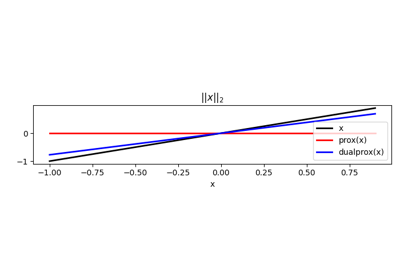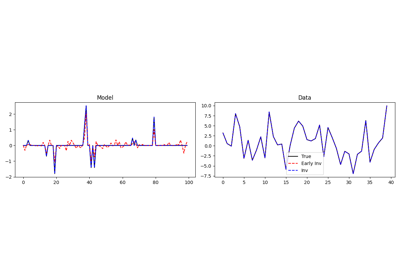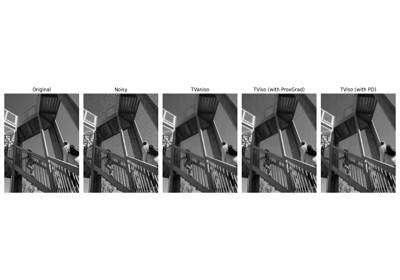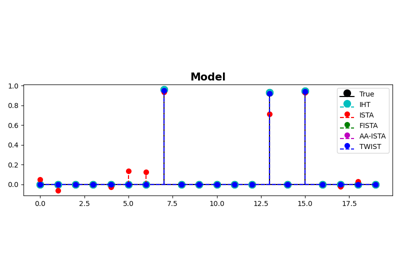# pyproximal.L1#

class pyproximal.L1(sigma=1.0, g=None)[source]#

L1 norm proximal operator.

Proximal operator of the $$\ell_1$$ norm: $$\sigma\|\mathbf{x} - \mathbf{g}\|_1 = \sigma \sum |x_i - g_i|$$.

Parameters
sigmafloat or list or np.ndarray or func, optional

Multiplicative coefficient of L1 norm. This can be a constant number, a list of values (for multidimensional inputs, acting on the second dimension) or a function that is called passing a counter which keeps track of how many times the prox method has been invoked before and returns a scalar (or a list of) sigma to be used.

gnp.ndarray, optional

Vector to be subtracted

Notes

The $$\ell_1$$ proximal operator is defined as :

$\begin{split}\prox_{\tau \sigma \|\cdot\|_1}(\mathbf{x}) = \operatorname{soft}(\mathbf{x}, \tau \sigma) = \begin{cases} x_i + \tau \sigma, & x_i - g_i < -\tau \sigma \\ g_i, & -\tau\sigma \leq x_i - g_i \leq \tau\sigma \\ x_i - \tau\sigma, & x_i - g_i > \tau\sigma\\ \end{cases}\end{split}$

where $$\operatorname{soft}$$ is the so-called called soft thresholding.

Moreover, as the conjugate of the $$\ell_1$$ norm is the orthogonal projection of its dual norm (i.e., $$\ell_\inf$$ norm) onto a unit ball, its dual operator (when $$\mathbf{g}=\mathbf{0}$$) is defined as:

$\begin{split}\prox^*_{\tau \sigma \|\cdot\|_1}(\mathbf{x}) = P_{\|\cdot\|_{\infty} <=\sigma}(\mathbf{x}) = \begin{cases} -\sigma, & x_i < -\sigma \\ x_i,& -\sigma \leq x_i \leq \sigma \\ \sigma, & x_i > \sigma\\ \end{cases}\end{split}$
1

Chambolle, and A., Pock, “A first-order primal-dual algorithm for convex problems with applications to imaging”, Journal of Mathematical Imaging and Vision, 40, 8pp. 120–145. 2011.

Methods

 __init__([sigma, g]) affine_addition(v) Affine addition chain(g) Chain grad(x) Compute gradient postcomposition(sigma) Postcomposition precomposition(a, b) Precomposition prox(*args, **kwargs) proxdual(**kwargs)

## Examples using pyproximal.L1#Norms

NormsBasis Pursuit

Basis PursuitDenoising

DenoisingISTA, FISTA, and TWIST for Compressive sensing

ISTA, FISTA, and TWIST for Compressive sensing